# Classification of Induction Motor Bearing Failures Through Retro-Propagation Neural Network Algorithm and Adaptive Neuro-Fuzzy Inference System of Type Takagi-Sugeno

Classification of Induction Motor Bearing Failures Through Retro-Propagation Neural Network Algorithm and Adaptive Neuro-Fuzzy Inference System of Type Takagi-Sugeno
Department of Electrical Engineering, Larbi Tebessi University, Tebessa 12000, Algeria

Mining Laboratory, Department of Electrical Engineering, University Badji Mokhtar, Annaba 23000, Algeria

LABGET Laboratory, Department of Electrical Engineering, Larbi Tebessi University, Tebessa 12000, Algeria

Corresponding Author Email:
bouguerneabla@yahoo.fr
Page:
149-154
|
DOI:
https://doi.org/10.18280/ejee.240304
13 February 2022
|
Accepted:
6 June 2022
|
Published:
30 June 2022
| Citation

OPEN ACCESS

Abstract:

The goal of this work was to study the best technique for fault diagnosis in bearing induction motors. Degraded operating modes may occur during the life of the induction motors. One of the main causes of these failures is the defects of the bearings. To improve the operational safety of the drives, monitoring facilities can be placed to perform preventive maintenance. We present a classification of the vibration vector signal based on the vibration data obtained from the vector signal for four types of bearing defects (healthy, ball defect, inner ring and outer ring defect). The automatic diagnosis of these vectors is performed using artificial intelligence techniques that combine retro-propagation neural network algorithm and fuzzy inference system adaptive network of type Takagi-Sugeno. These techniques give accurate results that are confirmed by numerical simulation.

Keywords:

classification, induction motor, bearing, artificial intelligence techniques, artificial neural networks, adaptive neuro-fuzzy inference system, Takagi-Sugeno

1. Introduction

Neural networks and fuzzy logic are two concepts totally inspired by human reasoning. Although these two models differ in structure, they have many similarities. Indeed, the use of these two techniques does not require a well-defined mathematical model since the resolution is based on the numerical values of inputs and outputs for a given neural network and on the logic of the neural network system in the case of fuzzy logic.

The common use of neural networks and fuzzy logic allows to obtain the benefits of both methods: The learning power of the first method, readability and flexibility of the second. They have given rise to neuro-nofluous systems, most often oriented towards complex problems of classification and control of systems [1, 2]. Figure 1 summarizes the principle of a fuzzy neural system representing the intersection of fuzzy logic and the neural network.

Various combinations of these two methods have been developed since 1988. The fuzzy neural system is defined as a multilayer neural network with fuzzy parameters, or as a fuzzy system applied in parallel distribution, many types of neuro-fuzzy systems have been studied. Identified and developed in recent years, far from unification, and sometimes equivalent and confusing .

The Hybrid Neural-fuzzy System is a neural network with a fuzzy signal, a fuzzy weights, a fuzzy transfer function and a module to adjust the structure of the fuzzy neural network by learning a data set [4, 5].

The fuzzy neural system refers to how to apply different learning techniques to develop documents ranging from the neural network to the fuzzy inference system. Several books and articles show good performance of the ANFIS model when used in engine control.

The current work is particularly concerned with the diagnosis and classification of mechanical failures of induction motors, in particular bearing failures (Outer race, Inner race and ball fault).

Therefore, the proposed approach relies on the artificial neural network and the adaptive fuzzy neural inference system for more effective and reliable induction motor diagnosis.

## 1.pngFigure 1. Principle of the fuzzy neuron system

2. Bearing Failures

The system for diagnosing mechanical failures of induction motors has also been discussed in the drive systems of induction motors [6, 7].

Examples of bearing failure, damage between insulation windings, stator current, rotor speed, motor winding temperature, engine rolling temperature and noise as input data for diagnostic technique assumptions . The approach proposed in this article uses vibration signal analysis to diagnose mechanical induction motors .

We are particularly interested in bearing failures (outer ring, inner ring and ball defect) of induction motors, as shown in Figure 2. While Table 1 detail the bearing (6205-2RS-JEM SKF) parameters.

## 2.png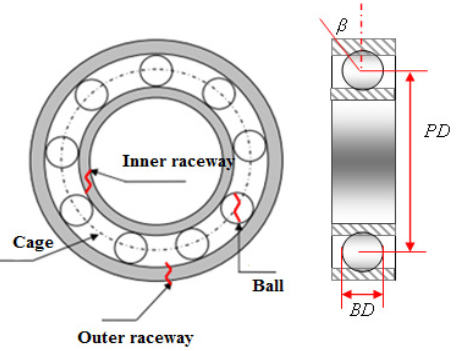Figure 2. Various failures of bearing

Table 1. Bearing parameters details (6205-2RS-JEM SKF)

 Parameters Values(mm) Inner Race Diameter 25.001 Outer Race Diameter 51.998 Thickness 15.001 Ball Diameter 7.940 Pitch Diameter 39.039
3. Artificial Neural Networks

In this type of learning, the adaptation of the convolution coefficients is not based on a comparison with a certain desired result, but on the network itself, which organizes the entries presented in such a way as to optimize a function at certain costs, without providing further elements of the desired response [10, 11].

The algorithm used to train the neural network is an error gradient back-propagation algorithm. Retro propagation is currently the most widely used tool in the field of neural networks. In general, the different stages of learning consist of four stages :

• Synaptic network weight initialization: In practice, this is done by small random values other than zero . This is a derivative calculation technique applicable to any structure of these discriminate functions.

In a network (s) layers with (n) inputs and (m) outputs, the state of individual neurons is given by:

$U_{i}^{l}(k)=f^{l}\left(P_{i}^{l}(k)\right)$                      (1)

with

$P_{i}^{l}(k)=\sum_{j=1}^{N_{l-1}} W_{i j}^{l} U_{j}^{l-1}(k)$                 (2)

where, i=1, 2, ..., Nl, j=1, 2, ..., Nl-1, q=1, 2, ..., Nl+1; N number of neurons in the layer l; Nl-1 number of neurons in layer l-1; Nl+1 Number of neurons in layer l+1; L The number of layers; $U_{i}^{l}(k)$ Output of neuron iof the layer l; $W_{i j}^{l}(k)$ Synaptic coefficient (weight) of the $j^{éme}$ input neuron (i) of the layer(s).

Input signal presentation: The input signal is presented at the input of the network; An actual output will be calculated. This calculation is performed close to the input layer towards the output layer, which is called the activation propagation.

$U_{i}^{0}(k)=X_{i}(k) \quad i=1,2, \ldots, n$                    (3)

$U_{i}^{0}(k)=Y_{i}(k) \quad i=1,2, \ldots, m$                      (4)

where, Xi(k) and Yi(k) are respectively the inputs and outputs of the network.

• Error calculation: In the case of supervised learning, the error will take into account the difference between the input signal and the reference signal .

• Calculation of correction vector: from the error vector, correction is applied to the synaptic weights of connections and the threshold of the neuron is determined .

The goal of the back-propagation method is to adjust the parameters $W_{i j}^{1}$ to minimize a cost function given by:

$E(W)=\sum_{P=1}^{T} E_{P}(W)$                  (5)

with

$E_{P}(W)=\frac{1}{2} \sum_{i=1}^{m}\left[y_{i}^{d}(k)-y_{i}(k)\right]^{2}$                   (6)

4. Adaptive Neuro-Fuzzy Inference System

Adaptive Neuro-Fuzzy Inference System (ANFIS) presents fuzzy inference system using association learning and adaptive networks are implemented (Figure 3) .

This architecture perfects fuzzy rules obtained by human experts to describe the input-output behavior of a complex system. Fuzzy neural inference systems combine the advantages of two complementary techniques .

## 3.png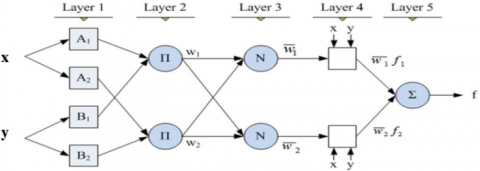Figure 3. Structure of the ANFIS

Fuzzy systems provide a good representation of knowledge. In particular, they are identified in three different families :

• FALCON model

• NEFCLASS model

• ANFIS model

It is assumed that there are two language input variables x and y and an F output, and the base rule contains two rules:

Rule1: if x is A1 and y is B1 THEN F1=p1 x+q1 y+r1

Rule2: if x is A2 and y is B2 THEN F2=p2 x+q2 y+r2

where, x and y are the input variables; A1, A2, B1 and B2 of the fuzzy assemblies, Fi the outputs of all the neurons of defuzzification; pi, qi and ri are parameters of the i rule’s consequence determined during the learning process.

First layer (Fuzzification)

Each node in this layer has a function such as :

$O_{i}^{1}=f_{i 1}(x)=\mu_{A i}(x)$                   (7)

where, x is the entry of the respective node i; Ai is the linguistic term associated with the function of belonging μAi; $O_{i}^{1}$ is the output of the first layer, therefore represents the degrees of belonging of the input variable x.

Second layer (Generation of the weight of rule i)

This layer is formed by circular nodes of fixed type are noted Π, each node corresponds to a fuzzy rule Sugeno. And each of them generates in output the product (operator AND fuzzy logic) of its inputs, and which corresponds to the degree of membership of the rule considered we have :

$W_{i}=\mu_{A i}(x) \cdot \mu_{B i-2}(y) y=1, 2$                   (8)

Third layer (Normalization of the weights of rule i)

The nodes of this layer are circular and also fixed called N, each node computes the normalized activation of a given fuzzy rule, depending on the relationship :

$V_{1}=W_{i} / W_{1}+W_{2} i=1, 2$                     (9)

All exits from this layer are called standard weights .

Fourth layer (Consequence)

Calculation of the exit of the rules where defuzzification

Each node in this layer is a square node with a function performing the following calculation:

$O_{i}^{4}=V_{i} \cdot f_{i}=V_{i} \cdot\left(P_{i} x+q_{i} y+r_{i}\right) i=1,2$                  (10)

where, Vi is the output of the third layer, and ri are the set of parameters designated under the consequent name.

Fifth layer (Summation)

Includes a single node that provides the output of ANFIS by calculating the sum of the outputs of all defuzzification nodes, i.e.:

$O_{i}^{5}=F \sum_{i} V_{i} \cdot f_{i}$                (11)

5. Experimental Results

The three-phase induction motor is connected to the dynamometer and torque sensor by an automatic alignment coupling method. The power scale is controlled at the required load.

The accelerometer is installed in the engine dormitory and receives the vibratory signal from the charger . The assembled data system consists of a broadband amplifier specially designed for vibration signals and a data logger with a specific sampling frequency. Many noise sensors are used for data recording because they are very reliable and more accurate. The bearings are installed in the mechanical transmission with the motor as shown in Figure 4 .

## 4.pngFigure 4. Experimental setup

We note that the seismic signal data analyzed for health and malfunctions are also Downloading via website: [http://www.eecs.cwru.edu/laboratory/bearing/download.htm].

5.1 Database analysis

For each case, the vibration signal analyzed is divided into four parts, each with a recording period, the energy is calculated and finally we obtain 16 elements of each vector. The procedure was repeated for all observed defects.

In addition, for each sub-category, the individual assigns a number that effectively represents the elements. From the output vector (decision), namely health status "0", Ball error case "1", internal error "2" and external error "3". Then we move on to learning each class of. By default and displays a vector whose size is reduced compared to the roof.

In the Bearing Data Centre, the Outer Ring or Inner Ring failure error signals and the Ball fault signals with an error size of 0.53 mm were collected and used in this study. Figure 5 shows the vibration signals of the three defects.

However, classes and tests apply; we have tested ANN and ANFIS so its effectiveness.

## 5.png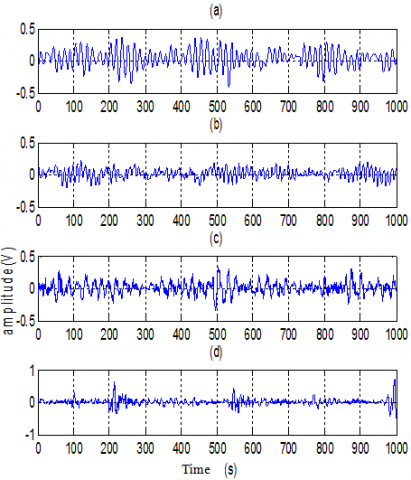Figure 5. Vibration database signals for (a) healthy machine, (b) inner race failure, (c) Ball failure, (d) outer race failure

5.2 Classification by Artificial Neural Networks (ANN)

This section presents the classification task to which the neural network is successfully applied. In the classification task, the output consists of Boolean functions that indicate whether the instance belongs to a class or not.

For the classification task, we want the neural network to give correct outputs on a set of examples, called the test set.

To do this, we will use a set of examples where the network will practice and learn. This set is called a learning set. Test set examples are not part of the learning set. The test set is much larger than the learning set.

The set of activities includes all the concrete examples encountered during the end-use of a neural network. With this method based on the distinction between learning and test sets, we see that the neural network must be able to generalize.

Figure 6 and Table 2 show that the integration of artificial neural networks into these bearing fault diagnostic systems is effective and that the mean square error is very low (MSE<<10-17). Thus, classification systems improve their performance thanks to the learning capacity of the neural network.

## 6.pngFigure 6. Mean square error

Table 2. Mean square error for four classes

 C1 C2 C3 C4 Decision Performance 0.2626 2.6971e-04 0.0129 0.1229 C1 7.5866 e-26 2.4335e-04 0.2161 0.0448 0.0077 C2 1.0969 e-17 4.7484e-04 0.0626 0.4007 0.1089 C3 7.2296 e-21 0.0561 0.0056 0.0977 0.2168 C4 7.3323 e-26

Note: C1 healthy machine, C2 inner race failure classes, C3 Ball failure classes, C4 outer race failure classes.

5.3 Classification by Neuro-Fuzzy system

The Adaptive Fuzzy Neural Inference System is one of the most comprehensive power methods for the automatic diagnosis of electrical machines and has been successfully applied in recent years.

Figure 7 shows the structure of the ANFIS and can be summarized in three steps, taken in sequence.

## 7.pngFigure 7. ANFIS steps

ANFIS (Adaptive Network-Based Fuzzy Inference System) is an adaptive neural-fuzzy inference system that uses a five layer MLP neural network where each layer corresponds to a single step of the Takagi-Sugeno fuzzy inference system.

This system is based on member functions and their rules. Mamdani and Assilian were inspired by Mohamed Zadeh for this logic, proposing a more widely used model called Mamdani.

Back Propagation of ANFIS

• The Error measurement Ek for kth learning data entry.

$E_{k}=\sum_{i=1}^{N(L)}\left(d_{i}-x_{L, i}\right)^{2}$                  (12)

where, N(L): Number of neurons in the layer L; di: Desired output vector component; xL,i: Real output vector component (from ANFIS).

• Global error measurement E:

$E=\sum_{k=1}^{K} E_{k}$                    (13)

• For each parameter $\alpha_{i}\left(\alpha_{i} \in S_{1}\right)$ are modified according to the equation:

$\Delta \alpha_{i}=-\eta \frac{d E}{d \alpha_{i}}$                 (14)

η is a positive constant called learning step.

The structure generated with the MATLAB interface is illustrated in Figure 8.

## 8.png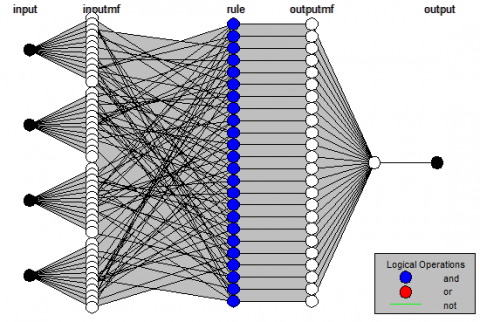Figure 8. ANFIS model structure

Figure 9 presents the results of fuzzy neural inference with the MATLAB interface.

## 9.png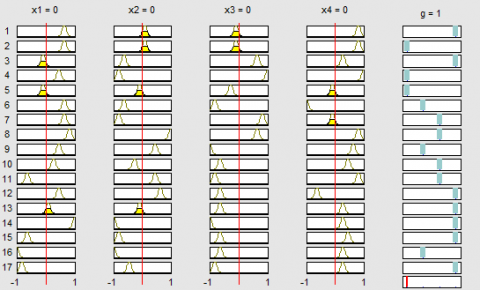Figure 9. Fuzzy-neuro inference

The ANFIS driven and verified outputs presented by learning and testing network for different types of error diagnosis are shown in Figures 10 and 11 respectively, resulting in negligible dispersion.

## 10.png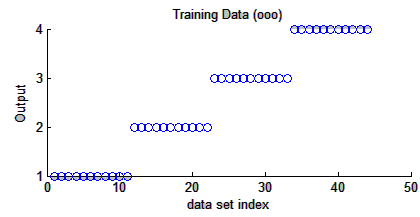Figure 10. Network learning

## 11.png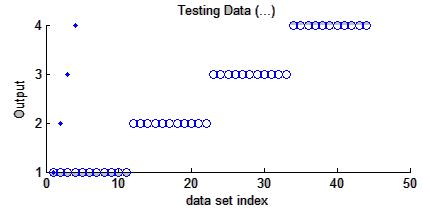Figure 11. Network test

Figure 12 shows a new vector error classifier from which we observed a good group. An improved ANFIS performance of more than 99% can be illustrated from this figure.

The square root of the square error and the error curve for formation are shown in Figure 13. In practice, the error is less than 1.5 %. The result turned out to be 0.25%. New actual data verified with ANFIS Model updated a classification error at 1.3754. The input or dependency and output relationships of ANFIS are also analyzed. These are the unique features of the adaptive fuzzy neural inference system. The ANFIS are considered excellent and effeteness technique classification failure in induction motor considering their performance.

## 12.png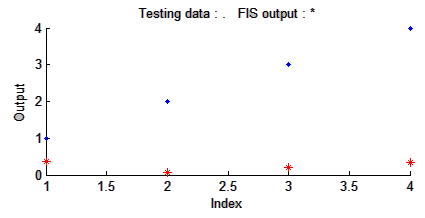Figure 12. Classification task

## 13.png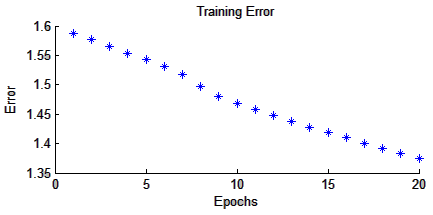Figure 13. Classification error

Unlike the neural network, the input and output designation in ANFIS is not a black box.

6. Conclusions

The integration of neural networks into these systems improves their performance through the learning capabilities of neural networks. On the other hand, the introduction of fuzzy rules in the neural network, often important because they are not easy to read, clarifies the meaning of the parameters of the network and facilitates their initialization; this represents a significant time saving for their identification.

The Retro-propagation neural network algorithm needs to know only specific cases or exceptions, not a complete problem. The advantage of the fuzzy-neuro strategy technique is that it uses the knowledge available through the rule base, reducing the size of the rule base: sufficient to have detailed and general rules to be provided by the neural network algorithm and reduce the complexity of learning. Immediate effectiveness starts of learning and potential to avoid initial erratic behaviors.

This model is made in the FUZZY-NEURO toolbox of MATLAB/SIMULINK. It gives very good results in tracking, non-linear approximation, dynamic control and signal processing.

References

 Bindu, S., Thomas, V.V. (2014). Diagnoses of internal faults of three phase squirrel cage induction motor—A review. In 2014 International Conference on Advances in Energy Conversion Technologies (ICAECT), pp. 48-54. https://doi.org/10.1109/ICAECT.2014.6757060

 Lei, Y., Jia, F., Lin, J., Xing, S., Ding, S.X. (2016). An intelligent fault diagnosis method using unsupervised feature learning towards mechanical big data. IEEE Transactions on Industrial Electronics, 63(5): 3137-3147. https://doi.org/10.1109/TIE.2016.2519325

 Lee, K.B., Cheon, S., Kim, C.O. (2017). A convolutional neural network for fault classification and diagnosis in semiconductor manufacturing processes. IEEE Transactions on Semiconductor Manufacturing, 30(2): 135-142. https://doi.org/10.1109/TSM.2017.2676245

 Lou, X., Loparo, K.A. (2004). Bearing fault diagnosis based on wavelet transform and fuzzy inference. Mechanical Systems and Signal Processing, 18(5): 1077-1095. https://doi.org/10.1016/S0888-3270(03)00077-3

 Zhao, R., Yan, R., Chen, Z., Mao, K., Wang, P., Gao, R.X. (2019). Deep learning and its applications to machine health monitoring. Mechanical Systems and Signal Processing, 115(15): 213-237. https://doi.org/10.1016/J.YMSSP.2018.05.050

 Jia, F., Lei, Y., Lin, J., Zhou, X., Lu, N. (2016). Deep neural networks: A promising tool for fault characteristic mining and intelligent diagnosis of rotating machinery with massive data. Mechanical Systems and Signal Processing, 72: 303-315. https://doi.org/10.1016/j.ymssp.2015.10.025

 Cho, H.C., Knowles, J., Fadali, M.S., Lee, K.S. (2009). Fault detection and isolation of induction motors using recurrent neural networks and dynamic Bayesian modeling. IEEE Transactions on Control Systems Technology, 18(2): 430-437. https://doi.org/10.1109/TCST.2009.2020863

 Lei, Y., Jia, F., Lin, J., Xing, S., Ding, S. (2016). An intelligent fault diagnosis method using unsupervised feature learning towards mechanical big data. IEEE Transactions on Industrial Electronics, 63(5): 3137-3147. https://doi.org/10.1109/TIE.2016.2519325

 Chen, C., Zhang, B., Vachtsevanos, G. (2012). Prediction of machine health condition using neuro-fuzzy and Bayesian algorithms. IEEE Transactions on Instrumentation and Measurement, 61(2): 297-306. https://doi.org/10.1109/TIM.2011.2169182

 Arel, I., Rose, D.C., Karnowski, T.P. (2010). Research frontier: deep machine learning–A new frontier in artificial intelligence research. IEEE Computational Intelligence Magazine, 5(4): 13-18. https://doi.org/10.1109/MCI.2010.938364

 Chen, X.W., Lin, X. (2014). Big data deep learning: challenges and perspectives. IEEE Access, 2: 514-525. https://doi.org/10.1109/ACCESS.2014.2325029

 Yang, X., Yan, R., Gao, R.X. (2015). Induction motor fault diagnosis using multiple class feature selection. Proceedings of 2015 IEEE International Instrumentation and Measurement Technology Conference (I2MTC), Pisa, Italy, pp. 256-260. https://doi.org/10.1007/s10033-017-0189-y

 Martínez-Morales, J.D., Palacios-Hernández, E.R., Campos-Delgado, D. (2018). Multiple-fault diagnosis in induction motors through support vector machine classification at variable operating conditions. Electrical Engineering, 100(1): 59-73. https://doi.org/10.1007/s00202-016-0487-x

 Liang, X, Edomwandekhoe, K. (2017). Condition monitoring techniques for induction motors. In: 2017 IEEE Industry Applications Society Annual Meeting, pp. 1-10. https://doi.org/10.1109/IAS.2017.8101860

 Liu, R., Yang, B., Zio, E., Chen, X. (2018). Artificial intelligence for fault diagnosis of rotating machinery: A review. Mechanical Systems and Signal Processing, 108: 33-47. https://doi.org/ 10.1016/J.YMSSP.2018.02.016

 Mohd Amiruddin, A.M., Zabiri, H., Taqvi, S.A., Tufa, L. (2020). Neural network applications in fault diagnosis and detection: An overview of implementations in engineering-related systems. Neural Computing and Applications, 32: 447-472. https://doi.org/10.1007/s00521-018-3911-5

 Seera, M., Lim, C. P., Ishak, D., Singh, H. (2013). Application of the fuzzy min–max neural network to fault detection and diagnosis of induction motors. Neural Computing and Applications, 23(1): 191-200. https://doi.org/ 10.1007/s00521-012-1310-x

 Su, H., Chong, K.T., Ravi Kumar, R. (2011). Vibration signal analysis for electrical fault detection of induction machine using neural networks. Neural Computing and Applications, 20(2): 183-194. https://doi.org/10.1007/s00521-010-0512-3

 Azgomi, H.F., Poshtan, J. (2013). Induction motor stator fault detection via fuzzy logic. In 2013 21st Iranian Conference on Electrical Engineering (ICEE), pp. 1-5. https://doi.org/10.1109/IranianCEE.2013.6599711

 Eldin, E.M.T., Emara, H.R., Aboul-Zahab, E.M., Refaat, S.S. (2007). Monitoring and diagnosis of external faults in three phase induction motors using artificial neural network. In 2007 IEEE Power Engineering Society General Meeting, pp. 1-7. https://doi.org/10.1109/PES.2007.385469

 Dias, C.G. (2018). A neuro-fuzzy approach for locating broken rotor bars in induction motors at very low slip. Journal of Control, Automation and Electrical Systems, 29(4): 489-499. https://doi.org/10.1007/s40313-018-0388-5

 Liang, X., Ilochonwu, O. (2010). Induction motor starting in practical industrial applications. IEEE Transactions on Industry Applications, 47(1): 271-280. https://doi.org/ 10.1109/TIA.2010.2090848

 Oong, T.H., Isa, N.A.M. (2011). Adaptive evolutionary artificial neural networks for pattern classification. IEEE Transactions on Neural Networks, 22(11): 1823-1836. https://doi.org/10.1109/TNN.2011.2169426

 Deng, Y., Ren, Z., Kong, Y., Bao, F., Dai, Q. (2016). A hierarchical fused fuzzy deep neural network for data classification. IEEE Transactions on Fuzzy Systems, 25(4): 1006-1012. https://doi.org/10.1109/TFUZZ.2016.2574915